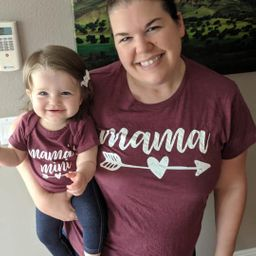Our Discord hit 10K members! 🎉 Meet students and ask top educators your questions.Join Here!Numerade Educator

# Find the mean, median, and mode.$$22,35,45,46$$

## mean: $37 ;$ median: $40 ;$ no mode

#### Topics

No Related Subtopics

### Discussion

You must be signed in to discuss.

### Video Transcript

well, first off with finding the mean, which is taking the sum of all of these numbers and then dividing by four. So the mean would be 1 48 after we add it divided by the number of items on our list, which is four. And that leaves us with 37. The mode would be the number that occurs the most amount of times. You can see that every number here occurs just once, so there is no mode the median will find by rearranging these into numerical order. Actually, they're already in the least two greatest order, so the media would be the number of directly in the middle. Since we haven't even number of items will take. The two middle 35 45 will add them together and divide by two. When we do that, we get 40 and thus are mediumOther Schools

#### Topics

No Related Subtopics